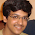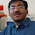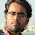### Increasing Sequence in Dice

Source: A book on probability puzzles

Problem:
Suppose we play a game with a die where we roll and sum our rolls as long as we keep rolling larger values. For instance, we might roll a sequence like 1-3-4 and then roll a 2, so our sum would be 8. If we roll a 6 first, then we’re through and our sum is 6.

(a) What is the expected value of the sum?

(b) What is the expected value of the number of rolls?

(c) If the game is played with an n-sided die, what happens to the expected number of rolls as n approaches infinity?

Update (Aug 31, 2011)
Solution posted by Gaurav Sinha (chera, IITK 1996 Graduate, Indian Revenue Service) and Siva in comments!

1.What if we get say:
1 1..

Will we continue or not?

2.a. Its 6 (=n)
b. for n its like (1+1/n)^(n-1)
c. e

Mostly used recursion.

care to enlighten us on the name of the puzzle book?

3.siva has already given answers for a general n-face die. for parts (b) & (c) i got slightly different answers.

i post the detailed solutions

part (a):

suppose S(r) is the expected sum if u get r on first roll.

in the next roll,if we get a number <=r then we stop otherwise sequence continues. pl. note we can get 1,2,...,n all with probability 1/n.

So, S(r)= r +(1/n)*( S(r) +S(r+1)+...+S(n)).

replacing r by r+1, we get
S(r+1)=r+1+(1/n)*(S(r+1)+...+S(n)).

substracting the above two equations, we get

S(r)= (1+1/n)* S(r+1)-1.

now we know that S(n)=n.

hence on repeated application of above recurrence , S(n-1)=...=S(1)=n.

the final answer we want is then

(1/n) sigma S(i), i= 1 to n.
=n as each S(i)=n.

Part (b): Similar to above. if we let X(r) be the expected number of rolls if we get r on first roll, then

X(r)=1+(1/n)*( X(r+1)+...+X(n)).
replacing r by r+1, and substracting the two equations, we get,
X(r)=X(r+1)(1+1/n).

X(n)=1.

So, X(r)= (1+1/n)^(n-r).

our final answer will then be

(1/n) sigma X(r), r= 1 to n.
=(1+1/n)^n -1

part(c): lim n->infinity,
(1+1/n)^n -1
=e-1.

the above answer to part (c) makes me wonder if there is some mistake.

4.a typo mistake in part (a) of my previous post: i repost entire solution.

part (a):

suppose S(r) is the expected sum if u get r on first roll.

in the next roll,if we get a number <=r then we stop otherwise sequence continues. pl. note we can get 1,2,...,n all with probability 1/n.

So, S(r)= r +(1/n)*(S(r+1)+...+S(n)).

replacing r by r+1, we get
S(r+1)=r+1+(1/n)*(S(r+2)+...+S(n)).

substracting the above two equations, we get

S(r)= (1+1/n)* S(r+1)-1.

now we know that S(n)=n.

hence on repeated application of above recurrence , S(n-1)=...=S(1)=n.

the final answer we want is then

(1/n) sigma S(i), i= 1 to n.
=n as each S(i)=n.

Part (b): Similar to above. if we let X(r) be the expected number of rolls if we get r on first roll, then

X(r)=1+(1/n)*( X(r+1)+...+X(n)).
replacing r by r+1, and substracting the two equations, we get,
X(r)=X(r+1)(1+1/n).

X(n)=1.

So, X(r)= (1+1/n)^(n-r).

our final answer will then be

(1/n) sigma X(r), r= 1 to n.
=(1+1/n)^n -1

part(c): lim n->infinity,
(1+1/n)^n -1
=e-1.

the above answer to part (c) makes me wonder if there is some mistake.

5.@chera.. your solution is correct. But there is a small mistake. Answer to part c) is e and not e-1. You made a minor mistake in calculating the limit because you did not keep the brackets correct.
Thanks.

@sumit.. it will not continue. the sequence has to be increasing.

6.@ pratik. i checked from the link u gave of book. i think, siva has made a bracketing mistake, and answers i gave r correct.

7.My mistake. Yes, your solution is right.
Siva, can you please check if there is a mistake in your solution. Thanks.

8.@chera and @pratik, I guess the solution by @siva is right for part b) and c). I did all this in a very similar way as @chera using recursion and got expected no. of rolls as (1+1/n)^(n-1) which goes to e as n->infinity. The only mistake @chera has done is while calculating the expectation, he has missed to add 1 to the expression as we are supposed to count the first role also. In other words.
If E(r)=Expected no of future rolls given that the last roll is r. So according to law of total expectation.
E( total no of rolls) = (1/n)Sum_{ r = 1 to n} (E(r)+1)= 1+(1/n)Sum_{ r = 1 to n } => E(r) = (1+1/n)^(n-1)